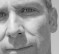## Decimate by M and Interpolate by L interchangeable if L and M are relatively primeStarted by 6 years ago6 replieslatest reply 6 years ago718 views

If you cascade an M-fold decimator and an L-fold interpolator, it is said to be equivalent to interchanging the order only if  L and M are relatively prime (GCD = 1). To that effect, there is an identity and a question in one of the textbooks as follows:

W = e^(-j2pi/M)

W^k and W^(kL) are equivalent only if L and M are relatively prime. Here, 0<= k <= M-1.

L is just a fixed integer, M is another fixed integer.

I've been trying a lot to come up with some scheme, but my numeric manipulation is very weak and I'm lost as to how to realize this proof. Can someone help to point me in the right direction as to how to think about such a problem?

[ - ]This looks like homework problem 4.7 on page 179 of Vaidyanathan's DSP book. My limited math skills prevent me from solving this problem, but I did have the following thoughts.

The problem is to show that the following S1 set of numbers is equal to the S2 set of numbers:

S1 = e-j2p0/M, e-j2p1/M, e-j2p2/M, ..., e-j2p(M-1)/M

S2 = e-j2p0L/M, e-j2p1L/M, e-j2p2L/M, ..., e-j2p(M-1)L/M

Looking at just the factors in the exponents, the problem becomes a problem in "number theory": show that the following S3 set of numbers is equal to the S4 set of numbers:

S3 = 0/M, 1/M, 2/M, ..., (M-1)/M

S4 = 0L/M, 1L/M, 2L/M, ..., (M-1)L/M

Because angles are periodic and the terms in S3 and S4 represent angular factors then those S3 and S4 numbers must be treated (manipulated) in modular arithmetic. That is, in modular arithmetic:

(M + 0)/M(mod M) = 0, (M + 1)/M(mod M) = 1, (M + 2)/M(mod M) = 2, and so on.

So it seems to me that the problem now is to prove:

The set of numbers kL/M(mod M) equals the set of numbers k/M,

for 0≤k≤M-1, and L and M are relatively prime. (Relatively

prime means that the ratio L/M cannot be simplified.)

I wonder if there's some identity in modulo number theory (congruence relations) that would help. Who knows?

Good Luck.

[ - ]Dr Mike and Rick,

Thank you so much for providing useful and clear explanations. I can now clearly see why this identity is true. Yes, this is precisely the problem 4-7 from P.P. Vaidyanathan's textbook. The explanation in the text in chapter 4 goes over this cascading of decimator and expander and leaves the proof to the reader and defers to problem 4-7.

So much to learn.. (patience, persistence, truth - dr. mike :-) )

Thank you!

[ - ]I think the easiest way to see this is to first assume L and M are not relatively prime.  Suppose L = rp and M = rs with p and s relatively prime.  Then e^(-j2pi L/M) = e^(-j2pi p/s) and the r cycle components do not appear.

If L and M are not relatively prime, k does not have to traverse 0 to M-1, it only has to go 0 to s-1.

I don't know if that is a valid "mathematical" proof, but it should give you a sense that some high level method can get there.

Patience, persistence, truth,

Dr. mike

[ - ]1- click on the 'thumbsup' button associated to the post(s) that you find helpful

2- click on the beer button to reward the users who helped you the most (no cost to you)

[ - ]